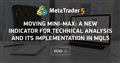# I try to make fib draw from last peak to last low and previous low to previous peak, but still compile "{" unexpected error. pls help.

```//+------------------------------------------------------------------+
//| Find Low                                                         |
//+------------------------------------------------------------------+
int FindLow(int mode, int count, int startBar) {

if (mode !=MODE_LOW && mode !=MODE_HIGH) return (-1);

int   currentBar = startBar;
int   foundBar  =  FindNextLow(mode, count*2+1, currentBar-count);
while (foundBar != currentBar) {
currentBar = FindNextLow(mode, count, currentBar+1);
foundBar = FindNextLow(mode, count*2+1, currentBar-count);

return (currentBar);

}

int FindNextLow(int mode, int count, int startBar) {

if (startBar<0) {
count     += startBar;
startBar   =0;

}
return( (mode==MODE_LOW) ?
iHighest(Symbol(), Period(), (MODE_HIGH)mode, count, startBar)  :
iLowest(Symbol(), Period(), (MODE_LOW)mode,count, startBar)
);

}

//+------------------------------------------------------------------+
//| Find Peak                                                        |
//+------------------------------------------------------------------+
int FindPeak(int mode, int count, int startBar) {

if (mode !=MODE_HIGH && mode !=MODE_LOW) return (-1);

int   currentBar = startBar;
int   foundBar  =  FindNextPeak(mode, count*2+1, currentBar-count);
while (foundBar != currentBar) {
currentBar = FindNextPeak(mode, count, currentBar+1);
foundBar = FindNextPeak(mode, count*2+1, currentBar-count);

}

return (currentBar);

}

int FindNextPeak(int mode, int count, int startBar) {

if (startBar<0) {
count     += startBar;
startBar   =0;

}
return( (mode==MODE_HIGH) ?
iHighest(Symbol(), Period(), (MODE_HIGH)mode, count, startBar)  :
iLowest(Symbol(), Period(), (MODE_LOW)mode,count, startBar)
)

}

//+------------------------------------------------------------------+
//| Expert initialization function                                   |
//+------------------------------------------------------------------+
int OnInit()
{
//---

//---
return(INIT_SUCCEEDED);
}
//+------------------------------------------------------------------+
//| Expert deinitialization function                                 |
//+------------------------------------------------------------------+
void OnDeinit(const int reason)
{
//---

}
//+------------------------------------------------------------------+
//| Expert tick function                                             |
//+------------------------------------------------------------------+
void OnTick()

{

int shoulder = 5;
int bar1;
int bar2;
int bar3;
int bar4;

bar1 = FindPeak(MODE_HIGH, shoulder, 1);
bar2 = FindPeak (MODE_HIGH, shoulder, bar1+1);
bar3 = FindLow(MODE_LOW, shoulder, 1);
bar4 = FindLow (MODE_LOW, shoulder, bar1+1);

ObjectDelete(0, "up");
ObjectCreate(0, "up",OBJ_FIBO, 0,  iTime(Symbol(), Period(), bar4), iHigh(Symbol(), Period(), bar4),iTime(Symbol(),Period(), bar1),iHigh(Symbol(), Period(),bar1));
ObjectSetInteger(0, "up", OBJPROP_COLOR, clrRed);
ObjectSetInteger(0, "up", OBJPROP_WIDTH, 3);

ObjectDelete(0, "down");
ObjectCreate(0, "down",OBJ_FIBO, 0,  iTime(Symbol(), Period(), bar2), iLow (Symbol(), Period(),bar2),iTime(Symbol(),Period(), bar3),iLow(Symbol(), Period(),bar3));
ObjectSetInteger(0, "down", OBJPROP_COLOR, clrRed);
ObjectSetInteger(0, "down", OBJPROP_WIDTH, 3);

}

```Moving Mini-Max: a New Indicator for Technical Analysis and Its Implementation in MQL5
• www.mql5.com
In the following article I am describing a process of implementing Moving Mini-Max indicator based on a paper by Z.G.Silagadze 'Moving Mini-max: a new indicator for technical analysis'. The idea of the indicator is based on simulation of quantum tunneling phenomena, proposed by G. Gamov in the theory of alpha decay.

Please edit your post and use the code button (Alt+S) when pasting code.
EDIT your original post, please do not just post the code correctly in a new post.

John Tsai:

```//+------------------------------------------------------------------+
//|                                                    test_fibo.mq5 |
//+------------------------------------------------------------------+
#property version   "1.00"
#property indicator_chart_window
//+------------------------------------------------------------------+
//| Custom indicator initialization function                         |
//+------------------------------------------------------------------+
int OnInit() {
//--- indicator buffers mapping

//---
return(INIT_SUCCEEDED);
}
//+------------------------------------------------------------------+
//| Custom indicator iteration function                              |
//+------------------------------------------------------------------+
int OnCalculate(const int rates_total,
const int prev_calculated,
const datetime &time[],
const double &open[],
const double &high[],
const double &low[],
const double &close[],
const long &tick_volume[],
const long &volume[],
//---

int shoulder = 5;
int bar1;
int bar2;
int bar3;
int bar4;

bar1 = FindPeak(MODE_HIGH, shoulder, 1);
bar2 = FindPeak (MODE_HIGH, shoulder, bar1+1);
bar3 = FindLow(MODE_LOW, shoulder, 1);
bar4 = FindLow (MODE_LOW, shoulder, bar1+1);

ObjectDelete(0, "up");
ObjectCreate(0, "up",OBJ_FIBO, 0,  iTime(Symbol(), Period(), bar4), iHigh(Symbol(), Period(), bar4),iTime(Symbol(),Period(), bar1),iHigh(Symbol(), Period(),bar1));
ObjectSetInteger(0, "up", OBJPROP_COLOR, clrRed);
ObjectSetInteger(0, "up", OBJPROP_WIDTH, 3);

ObjectDelete(0, "down");
ObjectCreate(0, "down",OBJ_FIBO, 0,  iTime(Symbol(), Period(), bar2), iLow (Symbol(), Period(),bar2),iTime(Symbol(),Period(), bar3),iLow(Symbol(), Period(),bar3));
ObjectSetInteger(0, "down", OBJPROP_COLOR, clrRed);
ObjectSetInteger(0, "down", OBJPROP_WIDTH, 3);
//--- return value of prev_calculated for next call
return(rates_total);
}
//+------------------------------------------------------------------+
//| Timer function                                                   |
//+------------------------------------------------------------------+
void OnTimer() {
//---

}
//+------------------------------------------------------------------+
//| ChartEvent function                                              |
//+------------------------------------------------------------------+
void OnChartEvent(const int id,
const long &lparam,
const double &dparam,
const string &sparam) {
//---

}
//+------------------------------------------------------------------+
//| Find Low                                                         |
//+------------------------------------------------------------------+
int FindLow(int mode, int count, int startBar) {

if (mode !=MODE_LOW && mode !=MODE_HIGH) return (-1);

int   currentBar = startBar;
int   foundBar  =  FindNextLow(mode, count*2+1, currentBar-count);
while (foundBar != currentBar) {
currentBar = FindNextLow(mode, count, currentBar+1);
foundBar = FindNextLow(mode, count*2+1, currentBar-count);

}
return (currentBar);
}
int FindNextLow(int mode, int count, int startBar) {

if (startBar<0) {
count     += startBar;
startBar   =0;

}
return( (mode==MODE_LOW) ?
iHighest(Symbol(), Period(), (MODE_HIGH), count, startBar)  :
iLowest(Symbol(), Period(), (MODE_LOW),count, startBar)
);

}
//+------------------------------------------------------------------+
//| Find Peak                                                        |
//+------------------------------------------------------------------+
int FindPeak(int mode, int count, int startBar) {

if (mode !=MODE_HIGH && mode !=MODE_LOW) return (-1);

int   currentBar = startBar;
int   foundBar  =  FindNextPeak(mode, count*2+1, currentBar-count);
while (foundBar != currentBar) {
currentBar = FindNextPeak(mode, count, currentBar+1);
foundBar = FindNextPeak(mode, count*2+1, currentBar-count);

}

return (currentBar);

}
//+------------------------------------------------------------------+
//| Find next Peak                                                        |
//+------------------------------------------------------------------+
int FindNextPeak(int mode, int count, int startBar) {

if (startBar<0) {
count     += startBar;
startBar   =0;

}
return( (mode==MODE_HIGH) ?
iHighest(Symbol(),Period(),(MODE_HIGH), count, startBar)  :
iLowest(Symbol(),Period(),(MODE_LOW),count, startBar)
);

}

//+------------------------------------------------------------------+
```

John Tsai: but still compile "{" unexpected error. pls help.

```int FindLow(int mode, int count, int startBar) {                     <<<< 1

if (mode !=MODE_LOW && mode !=MODE_HIGH) return (-1);

int   currentBar = startBar;
int   foundBar  =  FindNextLow(mode, count*2+1, currentBar-count);
while (foundBar != currentBar) {                                  <<<< 2
currentBar = FindNextLow(mode, count, currentBar+1);
foundBar = FindNextLow(mode, count*2+1, currentBar-count);
<<<<< ??????
return (currentBar);
}                                                                    <<<< 1

int FindNextLow(int mode, int count, int startBar) {```

Arpit T #:
Thank you

Hi

Yes – look on the braces
But also - if this is the mt5 code I think the iHighest and iLowest functions cannot be used in the same way as in mq4.

At least you need to set the direction of data series to get proper results. So check this out.

: But also - if this is the mt5 code I think the iHighest and iLowest functions cannot be used in the same way as in mq4.

Both on MQL4 and MQL5, iHighest and iLowest work in the same way. These functions were added to MQL5 at a later date, specifically to be compatible with MQL4.